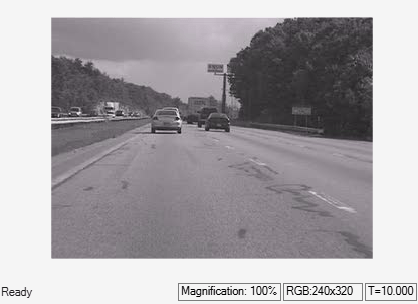# Import Video from MATLAB Workspace

This example shows how to import a video from MATLAB to Simulink workspace by using `Video` `From` `Workspace` block.

Load the video data to MATLAB workspace.

```load('videosignal.mat') ```

```modelname='ex_blkvideofromworkspace.slx'; open_system(modelname); ```The model reads the video data from MATLAB workspace. Set the `Form` `output` `after` `final` `value` `by` parameter of the `Video` `From` `Workspace` block to `Holding` `final` `value`. This parameter setting repeats the last frame of the video after generating all the frames.

Simulate the model and display the imported video signal by using `Video` `Viewer` block.

```sim(modelname); ```